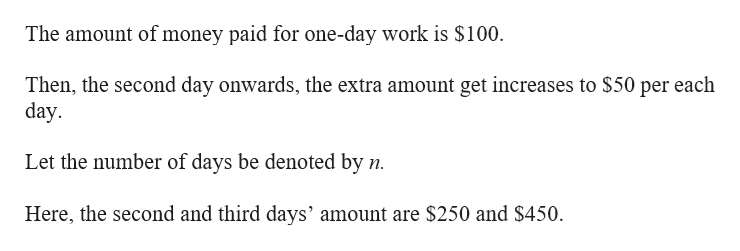# If you work one day, you earn \$100. Then you get a raise of \$50 each day you work. So if you work two days, you make a total of 250 (\$100+150). If you work three days, you a total of \$450. Write an equation.

Question
6 views

If you work one day, you earn \$100. Then you get a raise of \$50 each day you work. So if you work two days, you make a total of 250 (\$100+150). If you work three days, you a total of \$450. Write an equation.

check_circle

Step 1help_outlineImage TranscriptioncloseThe amount of money paid for one-day work is \$100 Then, the second day onwards, the extra amount get increases to \$50 per each day Let the number of days be denoted by n Here, the second and third days' amount are \$250 and \$450 fullscreen

### Want to see the full answer?

See Solution

#### Want to see this answer and more?

Solutions are written by subject experts who are available 24/7. Questions are typically answered within 1 hour.*

See Solution
*Response times may vary by subject and question.
Tagged in

### Other